# Regression vs Classification, Explained

This tutorial will quickly explain the difference between regression vs classification in machine learning.

I’ll explain what regression is, what classification is, and then compare them so you can understand the difference.

If you want to understand something specific, you can click on any of these links. They’ll take you to the proper section in the article.

As always though, you’ll probably gain a better understanding if you read the whole article from start to finish.

That said, let’s jump in.

## Regression vs Classification, a Quick Introduction

When you’re thinking about machine learning processes, it helps to break the process down into different parts. This can help you understand what’s actually going on.

Remember that machine learning is a process that enables a computer to learn from data.

To paraphrase Peter Flach in his book Machine Learning, machine learning is the creation of systems that improve their performance on a task with experience.

So when we do machine learning, there are a few parts of the process:

• the experience (i.e., the dataset)
• the algorithm (which experiences the data and performs on the task)
• the performance measure

Why does this matter?

Regression and classification are types of machine learning tasks.

Additionally, the structure of the input data (i.e., the “experience” that we use to train the system) is different in regression vs classification.

So to understand the difference between regression vs classification, it helps to understand the task that we’re trying to perform as well as the format of the input data.

Let’s take a look at both. We’ll start by distinguishing a regression task from a classification task.

## Regression and Classification are Machine learning Tasks

As I just mentioned, regression and classification are both types of machine learning tasks.

You can think of a task as the thing that the machine learning system is supposed to do.

When we build a machine learning system, we’re typically trying to do something. We’re trying to solve some sort of problem or accomplish something using a data-driven computer system.

When a machine learning system “learns”, it increases its ability to perform on the task.

### There are many types of machine learning tasks

So what types of things can machine learning tasks do?

In their book Deep Learning, Goodfellow, Bengio, and Courville describe a variety of tasks that machine learning systems can accomplish.

They start by explaining a fairly intuitive example. Let’s say that you wanted to build a robotic system that could walk. Walking is the task. There are a variety of ways we could solve this problem with a computerized system (different algorithms, etc), but the task is walking.

Moving away from robotics specifically and to machine learning generally, there are other types of tasks.

What if you wanted to build a computer system that could distinguish between cats and dogs? Distinguishing between cats and dogs is a task. Specifically, this is a classification task.

And there are a variety of other types of common tasks in machine learning:

• machine translation
• transcription
• denoising
• missing value imputation
• anomaly detection
• density estimation

… and a variety of others.

But perhaps the most common, and most important machine learning tasks – especially for beginners – are regression and classification.

Let’s look at regression and classification and see how they compare to eachother as machine learning tasks. After we do that, we’ll look at how they’re different from the perspective of the input data.

## Classification Predicts a Class, Regression Predicts a Number

One of simplest ways to see how regression is different from classification, is to look at the outputs of regression vs classification.

Put simply:

• In a regression task, we’re trying to predict a number
• In a classification task, we’re trying to predict a class

So in these two different tasks, the output of the system is different.To understand this better, let’s consider an example.

Let’s say that you’re trying to build a system that can distinguish between cats and dogs. That’s classification.

But let’s say that you have another task where you’re trying to predict house sale price in a particular city. That’s regression.

This is somewhat imprecise, but general rule of thumb is:

• If the output variable is numeric then it’s a regression problem
• If the output variable is categorical then it’s a classification problem

There are some exceptions to this, but that will help you understand the general difference between regression vs classification.

To help you understand these two machine learning tasks better though, it also helps to look at the format of the input data.

That let’s look at regression and classification from the input data perspective.

### Categorical data vs continuous data

One of the other ways to understand the difference between regression and classification is to look at the data that we use when we perform these tasks.

In order to do this, we first need to quickly review the difference between supervised learning and supervised learning.

It’s relevant when we’re talking about both regression and classification.

#### In Supervised Learning, We have a Target variable

Typically, regression and classification are both forms of supervised learning.

In supervised learning, we have a dataset that contains a set of input variables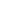,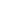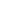. But in supervised learning, there is also a so-called “target” variable,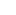.

This is in contrast to unsupervised learning, where we have input variables,,, but the target variableis absent.When we do supervised learning, we use a machine learning algorithm to build a machine learning model. The machine learning model “learns” to predict the outputbased on the input variables,.

Again, both regression and classification are forms of supervised learning, so the datasets for regression and classification problems both have a target variable,.

But, the exact form of the target variable is different for regression and classification.

### Regression Uses Continuous Data, Classification Uses Categorical Data

So how is the target variable different for regression and classification?

It’s fairly simple:

In regression, the target variable is continuous. Perhaps a different way to say this is that in regression, the target variable is a numeric variable. In regression, the values of the target variableare numbers.

But in classification, the target variable is categorical. In classification, the values of the target variableare categories. Typically, in classification, we call the value in the Y variable the label.So let’s think through the machine learning process for each different type of task, with their respective input data.

#### Classification Has Categorical Labels

When we use classification, we feed training data into a machine learning algorithm. The training data for classification has labels in thevariable.

As it’s exposed to examples (i.e., rows of data), the algorithm learns to predict thelabel based on the input values in the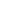variables. Classification algorithms learn how to predict a label based on input data.

Then later, when we give the algorithm unlabeled data (i.e., rows of data where thevalue is unpopulated), the algorithm can predict the label based only on the input values.

In classification, the algorithm trains on data that has categorical labels, and learns to predict categorical labels.

#### Regression has Numeric Target Values

For regression, the training data will have numeric target values populated for thevariable.

The algorithm learns to predict the numericvalue based on the input variables.

Then, when we later give the regression model new data that only has thevalues, the model will be able to predict the numericon the basis of thevalues.

In regression, the algorithm trains on data that has numeric target values, and learns to predict numeric target values.

## But, It’s Complicated

So at a high level, there are some simple ways that regression and classification are different from each other.

Having said that, it’s not always so clear cut. Sometimes, we use regression-like techniques to classify data. And visa versa.

Take for example, logistic regression. In logistic regression, the output is a 0 or a 1. In logistic regression, the output is a number. So technically, logistic regression is a form of regression.

BUT, when we actually use logistic regression, we almost always use it as a classifier. How? Let’s say that you want make a model that predicts whether a person will buy a particular product. The possible output categories would be “buy” and “no buy”. But if we recode “buy” as 1 and “no buy” as 0, we can apply logistic regression.

So by re-coding the target variable of our data, we can use logistic regression as a classifier. This is almost always how logistic regression is used, so it’s typically categorized as a classification technique, even though it’s technically a “regression” technique.

The point, is that there are some grey areas between regression and classification. It’s not always clear cut.

Do you still have questions about the difference between regression vs classification?

Are you confused about something, or do you think that I missed something?

If so, leave your questions in the comments section near the bottom of the page.

## There’s a lot more to learn

This article should have given you a good overview of regression vs classification.

But if you really want to master machine learning there is a lot more to learn. You’ll need to understand:

• data cleaning for machine learning
• model building
• model evaluation
• deployment

…. as well as a variety of specific machine learning techniques.

If you’re interested in learning more about machine learning, then sign up for our email list. Through this year and into the foreseeable future, we’ll be posting detailed tutorials about different parts of the machine learning workflow.

We plan to publish detailed tutorials about the different machine learning techniques, like linear regression, logistic regression, decision trees, neural networks, and more.

So if you want to master machine learning, then sign up for our email list. When you sign up, we’ll send our new tutorials directly to your inbox as soon as they’re published.

Joshua Ebner

Joshua Ebner is the founder, CEO, and Chief Data Scientist of Sharp Sight.   Prior to founding the company, Josh worked as a Data Scientist at Apple.   He has a degree in Physics from Cornell University.   For more daily data science advice, follow Josh on LinkedIn.

### 1 thought on “Regression vs Classification, Explained”

1. Please, give us a real example with a few real data using python. Thanks in advance.

Glob